## Introduction:

Survival Analysis is a Univariate Statistical technique in determining failure times(response variable) with lots of covariates. This technique is an extension of Logistic Regression, because there is a possibility of censoring happening in the data. Censoring is the condition where the failure time was failed to be observed at the end of the defined period of observation. There are two different types of censoring, depending on how the investigator define the period of observation. In type 1 censoring, the investigator define period of observation as a fixed value. In type 2 censoring, the investigator define period of observation as a random variable after d number of failures. The basic goal of survival analysis is to estimate and to interpret survivor function or hazard function, to compare hazard and/or survivor function, and to assess the relationship between covariates to survival time.

The main goal of this paper is to inform the reader about some Basic Survival Analysis techniques and to apply that technique in a small dataset setting.

# Distribution of Failure Times

Distribution of Failure Times is an important aspect of survival analysis. In order to correctly make an inference about survival data, it is necessary to obtain the exact distribution in which the survival data belong.

Common Vocabulary used in Distribution of Failure Times:

1.Survivor Function Survivor Function S(t) is the probability that a person survive longer than the specified time. The Survival Function is fundamental to Survival Analysis. This is often expressed by Kaplan Meier Curve. This function can be thought as the complement of cumulative density function.

$$S(t) = P(T>t) = 1- P(T \le t)$$

where T is RV

t is specified period of observation.

2. Probability Density Function Since most of the survival analysis case, we deal with continuous distribution, we can derive Probability Density Function $$f_{t}(t)$$ from the survivor function.

$f_{t}(t)= -S'(t)= \lim_{\delta \to 0^+} P(t \le T < t+\delta)$ to recover the survivor function, it is always possible to take the integral of probability density function.

3.Hazard Function Hazard Function denoted as $$h(t)$$ is defined as instantaneous potential per unit time for the event to occur given that the individual has survived up to time t. In contrast to the Survivor Function, hazard function focuses on the event of failing. Depending on the Failure Event that was defined, on average the higher hazard function, the worse the impact on the survival. Since Hazard function is defined as a rate rather than probability, the values of hazard function range between zero and infinity.

According to Cox and Oakes, knowing the survivor function is sufficient enough to be able to derive Hazard Function.

$h(t) = \lim_{\delta \to 0} P(t\le T < t+\delta|T>t)/ \delta$

by definition of conditional probability.

$h(t) = \lim_{\delta \to 0} P((t\le T < t+\delta) n (T > t))/ \delta *P(T>t)$

$h(t) = \lim_{\delta \to 0} P(t\le T < t+\delta)/\delta)/P(T>t)$

By definition, hazard function is just pdf divided by survivor function.

$h(t) = f_{t}(t) / S(t)$

where f(t) is defined as Probability density function, and S(t) is defined as survivor function.

These properties above will be useful in assessing distributional form of survival data. Look at appendix 2.2 of Cox and Oakes for more detail.

# Parametric Statistical Analysis:

From Survivor Function, and Hazard Function, it is possible to recover the survival distribution and gave some criteria for the appropriate choice of family.

Suppose that the specific family is selected and so the distribution is known up to a vector parameter of big omega a single sample of failure time.

Often, big omega transpose is written as (small omega transpose, and lambda transpose) where small omega transpose represent important parameter of survival distribution, and lambda transpose is just a noise parameter.

There are some theoretical aspect of Parametric Statistical Analysis that would be discussed in this part of the paper.

The Likelihood Function Survival Distribution has two components in it. it has continuous distribution until the period of observation and the contribution of the censored. That is to say that if the event happen to the subject before the period of observation ends, that particular subject only contributes to the continuous distribution. However, if the subject survived longer than the period of observation, that particular subject contributes to both of the continuous distribution and the censored distribution.

the full likelihood for n-independent subjects, indexed by i

$lik = \prod_{u} {f(ti;\phi)} * \prod_{c} {S(ci;\phi)}$

usually, it is easier to take the log transformation of likelihood, because the multiplication will become summation.

$l= \sum_{u} \log{f(ti;\phi)} + \sum_{c} \log{f(ti;\phi)}$

to get the maximum likelihood of phi, just differentiate the log-likelihood with respect to phi, and set it equal to 0.

The Major difference between Parametric Survival Model and the Proportional Hazard model is the distribution for survival time. In the Parametric Survival Model the distribution for survival time is known, and it follows certain distribution with unknown parameter. The proportional Hazard model that will be discussed in the subsequent part of this paper, does not have distribution for survival time. In fact the baseline model of proportional hazard model remains unknown.

# Kaplan Meier Survival Curve

Kaplan Meier method is a way to estimate and graph survival curve using product limit formula.

General KM formula: $$S(t_{f-1}) * P(T>T_{f}|T \ge t_{f})$$

where $$S (t_{f-1}) = \prod_{i=1}^{f-1} P(T>t_{f}|T\ge t_{f})$$

General formula for Kaplan Meier gives the probability of surviving past the previous failure times multiplied by the conditional probability of surviving past failure time, given survival function to at least that failure time.

The formula above can be proven using basic rule of conditional probability.

The main advantage of Kaplan Meier survival curve is to visually see the impact of treatment as oppose to the control group. In addition to that, if the experiment consists of multiple treatment, the Experimenter may be able to visually see which treatment is the best for the patients.

# Log Rank Test:

Log Rank Test is used to formally test that the true population survival curve is different. This is very similar to testing chi-square test of homogeneity. That is to say if the true population of survival curve is significantly different, we would expect that the proportion of survival group in treatment to be different than the proportion of survival group in control group.

When two groups are present for comparison, it is approximately distributed at chi-square with (2-1) df. The chisquare distribution with 1 df, is just a (standard normal)^2 distribution.

therefore $$\mbox {log-rank test} = (O_2- E_2)^2 / var(O_2 - E_2)$$

When more than two groups are present for comparison, it is approximately distributed at chi-square (G -1) df. Usually computer software is used to do exact log rank test calculation. However, it can also be approximated using $$\sum_{ij} (O_{ij}-E_{ij})^2/ E_{ij}$$ and compare the result with chi-square table to get the p-value.

If the p-value is less than specified $$\alpha$$, then we have enough evidence that the population survival curve are different. However, if the p-value is greater than the specified $$\alpha$$, then we do not have enough evidence to show that population survival curve are different.

There are different variations of Test Statistic to determine population survival curve, depending on the weight of Test Statistic. This determination of weight, should come from prior information on which part of survival curve is more important. This is where Bayesian point of view is being used in Survival Analysis.

Here is the example of log rank test being used in small dataset:

# Analysis on small dataset of survival data done in R

leukemia_patient=read.csv("leukemia.csv",header=T)
leukemia_patient
##    Time event Treatment
## 1     6     1      6-MP
## 2     6     1      6-MP
## 3     6     1      6-MP
## 4     6     0      6-MP
## 5     7     1      6-MP
## 6     9     0      6-MP
## 7    10     1      6-MP
## 8    10     0      6-MP
## 9    11     0      6-MP
## 10   13     1      6-MP
## 11   16     1      6-MP
## 12   17     0      6-MP
## 13   19     0      6-MP
## 14   20     0      6-MP
## 15   22     1      6-MP
## 16   23     1      6-MP
## 17   25     0      6-MP
## 18   32     0      6-MP
## 19   32     0      6-MP
## 20   34     0      6-MP
## 21   35     0      6-MP
## 22    1     1   Placebo
## 23    1     1   Placebo
## 24    2     1   Placebo
## 25    2     1   Placebo
## 26    3     1   Placebo
## 27    4     1   Placebo
## 28    4     1   Placebo
## 29    5     1   Placebo
## 30    5     1   Placebo
## 31    8     1   Placebo
## 32    8     1   Placebo
## 33    8     1   Placebo
## 34    8     1   Placebo
## 35   11     1   Placebo
## 36   11     1   Placebo
## 37   12     1   Placebo
## 38   12     1   Placebo
## 39   15     1   Placebo
## 40   17     1   Placebo
## 41   22     1   Placebo
## 42   23     1   Placebo
library(splines)
library(survival)
attach(leukemia_patient)
summary(Treatment)
##    6-MP Placebo
##      21      21

Log-Rank-Test

log_rank=survdiff(Surv(leukemia_patient$Time,leukemia_patient$event)~leukemia_patient$Treatment,rho=1) log_rank ## Call: ## survdiff(formula = Surv(leukemia_patient$Time, leukemia_patient$event) ~ ## leukemia_patient$Treatment, rho = 1)
##
##                                     N Observed Expected (O-E)^2/E
## leukemia_patient$Treatment=6-MP 21 5.12 12.00 3.94 ## leukemia_patient$Treatment=Placebo 21    14.55     7.68      6.16
##                                    (O-E)^2/V
## leukemia_patient$Treatment=6-MP 14.5 ## leukemia_patient$Treatment=Placebo      14.5
##
##  Chisq= 14.5  on 1 degrees of freedom, p= 0.000143

The P-value obtained from this log rank test is significantly small, indicating that the survival curve is significantly different between 6-MP group and Placebo group.

# Cox-Proportional Hazard Model

Cox-Proportional Hazard Model is o semiparametric hazard model. This proportional hazard model has two major components in the equation

$$\mbox h(X,T) = h_0 (t) * exp (\sum_i(\beta i*Xi))$$ Where $$h_o(t)$$ is the baseline hazard, and $$exp (\sum_i(\beta i*Xi))$$ is the exponential term. The major advantage of Cox-Proportional hazard model is that the baseline hazard does not need to be specified/semiparametric. In addition to that, Cox-Proportional Hazard Model has the robust estimate to the baseline hazard. In addition to that the exponential term of this Cox-Proportional Hazard Model will ensure that the Hazard function will always be non-negative.

One of the assumption of Cox-Proportional Hazard model is the time-independent covariates, or the covariates must not be changing over time. The Covariates like smoking status will easily failed this assumption, because people can change their smoking habit. For this reason, there are extension of this cox-proportional hazard model using joint probability technique.

Going back to Cox-Proportional Hazard Model. Usually, there are Three Statistical Objectives in Cox-Proportional Hazard model: 1. Test for significance of the effect. 2. Point estimate of the effect 3. Convidence-Interval of the effect.

## Testing for the Significance of the Effect:

There are two different Test Statistic used to test for the significance of the effect. The first test is Z-Statistic or commonly known as Wald Statistic. In this Test Statistic, Z was obtained from dividing coefficient with the corresponding Standard Error. The p-value from this Test Statistic was obtained from Z-table. If the p-value < alpha, then the effect of this particular covariate is significant.

Another Test Statistic that is commonly used to test for the significance of the effect is likelihood ratio test. This test makes use of the log-likelihood statistic obtained and multiply is by -2, and compared it with chi-square distribution with group-1 degree of freedom. If the p-value is less than alpha, then the effect of this particular covariate is significant.

Usually, Likelihood Ratio has better Statistical property, due to uniformly most powerful test by Neyman-Pearson Lemma. Therefore, when in doubt, Statistician usually used Likelihood Ratio Test.

## Point Estimate of the Effect:

In the experimental Design point of view, the point estimate of the effect is usually correspond to the least square estimate of the coefficient. Under normal distribution, the least square estimate was obtained from taking maximum-likelihood estimation of the coefficient. In Survival Analysis point of view, the point estimate of the effect is usually correspond to the hazard ratio. Similar to Odds Ratio, Hazard Ratio of 1 indicate that there is no different between treatment group and control group. Hazard Ratio that is greater than 1, indicate that the Treatment Group has more hazard than the Control Group. Hazard Ratio that is less than 1, indicate that the treatment group has less hazard than the control group.

## Confidence Interval of the effect:

Confidence Interval of the effect is usually one of the main statistical objectives in cox-proportional hazard model. Knowing Confidence Interval of the effect can also test whether or not that covariates is significant in determining survival time. Confidence Interval of the effect varies depending on whether or not the interaction between covariates is significant. If the interaction of covariates is not significant, then it is easier to model the 95% confidence interval.

$\mbox {95% CI for HR} = exp[\beta_i\pm 1.96\sqrt(var(\beta_i))]$

the sd($$\beta_i$$) = $$S\beta_i$$

if The interaction between covariates is significant, then the 95% CI of HR is slightly changes.

It is necessary to defined a term $$l= \beta_i +\sum_i (\beta_i*Wi)$$ where Wi correspond to the significant interaction between covariates.

$\mbox {95% CI of HR} = exp[l \pm1.96\sqrt(var(\beta_i))]$

Usually, the calculation of Confidence Interval of the effect is done in Statistical Software such as R, or SAS.

# An attempt to use Cox-Proportional Hazard Model to determine the effect of treatment

response_variable=Surv(leukemia_patient$Time,leukemia_patient$event)
surv_model=coxph(response_variable~Treatment)
summary(surv_model)
## Call:
## coxph(formula = response_variable ~ Treatment)
##
##   n= 42, number of events= 30
##
##                   coef exp(coef) se(coef)    z Pr(>|z|)
## TreatmentPlacebo 1.572     4.817    0.412 3.81  0.00014 ***
## ---
## Signif. codes:  0 '***' 0.001 '**' 0.01 '*' 0.05 '.' 0.1 ' ' 1
##
##                  exp(coef) exp(-coef) lower .95 upper .95
## TreatmentPlacebo      4.82      0.208      2.15      10.8
##
## Concordance= 0.69  (se = 0.053 )
## Rsquare= 0.322   (max possible= 0.988 )
## Likelihood ratio test= 16.4  on 1 df,   p=5.26e-05
## Wald test            = 14.5  on 1 df,   p=0.000138
## Score (logrank) test = 17.2  on 1 df,   p=3.28e-05

# Analysis part II

surv_model_2=survreg(response_variable~leukemia_patient$Treatment,dist="exponential") summary(surv_model_2) ## ## Call: ## survreg(formula = response_variable ~ leukemia_patient$Treatment,
##     dist = "exponential")
##                                   Value Std. Error     z        p
## (Intercept)                        3.69      0.333 11.06 2.00e-28
## leukemia_patient$TreatmentPlacebo -1.53 0.398 -3.83 1.27e-04 ## ## Scale fixed at 1 ## ## Exponential distribution ## Loglik(model)= -108.5 Loglik(intercept only)= -116.8 ## Chisq= 16.49 on 1 degrees of freedom, p= 4.9e-05 ## Number of Newton-Raphson Iterations: 4 ## n= 42 surv_model_3=survfit(response_variable~leukemia_patient$Treatment)
surv_model_3
## Call: survfit(formula = response_variable ~ leukemia_patient$Treatment) ## ## records n.max n.start events median ## leukemia_patient$Treatment=6-MP         21    21      21      9     23
## leukemia_patient$Treatment=Placebo 21 21 21 21 8 ## 0.95LCL 0.95UCL ## leukemia_patient$Treatment=6-MP         16      NA
## leukemia_patient$Treatment=Placebo 4 12 # Analysis part III surv_new= expand.grid(Treatment=c("6-MP","Placebo")) t(surv_new) ## [,1] [,2] ## Treatment "6-MP" "Placebo" surv_fit_1=survfit(surv_model,surv_new) surv_fit_1 ## Call: survfit(formula = surv_model, newdata = surv_new) ## ## records n.max n.start events median 0.95LCL 0.95UCL ## 1 42 42 42 30 23 16 NA ## 2 42 42 42 30 8 5 12 summary(surv_fit_1) ## Call: survfit(formula = surv_model, newdata = surv_new) ## ## time n.risk n.event survival1 survival2 ## 1 42 2 0.983 0.9227 ## 2 40 2 0.966 0.8453 ## 3 38 1 0.956 0.8067 ## 4 37 2 0.937 0.7293 ## 5 35 2 0.915 0.6520 ## 6 33 3 0.880 0.5415 ## 7 29 1 0.869 0.5077 ## 8 28 4 0.818 0.3794 ## 10 23 1 0.803 0.3468 ## 11 21 2 0.771 0.2849 ## 12 18 2 0.731 0.2216 ## 13 16 1 0.708 0.1899 ## 15 15 1 0.685 0.1620 ## 16 14 1 0.659 0.1341 ## 17 13 1 0.633 0.1101 ## 22 9 2 0.554 0.0580 ## 23 7 2 0.445 0.0202 plotstuff=expand.grid(line=c("solid"), colour=c("red","blue"),stringsAsFactors=F) plotstuff ## line colour ## 1 solid red ## 2 solid blue # Evaluating Cox-Proportional Hazard Model Suppose that an experimenter wants to know whether Cox-Proportional Hazard model. It is possible to do Goodness of fit to test whether or not Cox-Proportional Hazard model is appropriate for the Survival Data Analysis. There are three ways to check the whether the survival data fit with Cox-Proportional Hazard model assumption. The first way is through the Graphical Representation of -ln(-ln(S)) or Kaplan-Meier Curve vs the estimated curve using Cox-Proportional Hazard model. In addition to the Graphical representation, it is also possible to do Goodness of fit test, or extended Cox-model. ## Graphical Method: ln(-ln(S)): This is the first way to check whether or not the covariates in the data violates Cox Proportional Hazard model. If the ln(-ln(S)) curves show non-parallel pattern on certain covariates, then that covariate violates the assumption of Cox-Proportional Hazard model. This approach is very subjective. In addition to that, the parallel ln(-ln(S)) does not necessarily mean that the covariates follow the Cox-Proportional hazard Model assumption. Here is the example of ln(-ln(S)) plot to assess whether the treatment follows proportional hazard assumption #try to plot log(-log(survival)) library(splines) library(survival) surv_model_3=survfit(response_variable~leukemia_patient$Treatment)
surv_model_3
## Call: survfit(formula = response_variable ~ leukemia_patient$Treatment) ## ## records n.max n.start events median ## leukemia_patient$Treatment=6-MP         21    21      21      9     23
## leukemia_patient$Treatment=Placebo 21 21 21 21 8 ## 0.95LCL 0.95UCL ## leukemia_patient$Treatment=6-MP         16      NA
## leukemia_patient$Treatment=Placebo 4 12 surv_model_4=summary(surv_model_3) surv_model_4$surv
##   0.85714 0.80672 0.75294 0.69020 0.62745 0.53782 0.44818 0.90476
##   0.80952 0.76190 0.66667 0.57143 0.38095 0.28571 0.19048 0.14286
##  0.09524 0.04762 0.00000
data_frame=data.frame(surv_model_4$strata,surv_model_4$time,surv_model_4$surv) names(data_frame)=c("treatment","time","survival") data_frame[10:15,] ## treatment time survival ## 10 leukemia_patient$Treatment=Placebo    3   0.7619
## 11 leukemia_patient$Treatment=Placebo 4 0.6667 ## 12 leukemia_patient$Treatment=Placebo    5   0.5714
## 13 leukemia_patient$Treatment=Placebo 8 0.3810 ## 14 leukemia_patient$Treatment=Placebo   11   0.2857
## 15 leukemia_patient$Treatment=Placebo 12 0.1905 treatment_1=data_frame[data_frame$treatment=="leukemia_patient$Treatment=6-MP",] placebo=data_frame[data_frame$treatment=="leukemia_patient$Treatment=Placebo",] plot(treatment_1$time,log(-log(treatment_1$survival)),xlab="remission time in weeks",ylab="log-log survival",xlim=c(0,40),col="red",type="l",lty="solid",main="log-log curves by treatment") par(new=T) plot(placebo$time,log(-log(placebo$survival)),axes=F,xlab="remission time in weeks",ylab="log-log survival",xlim=c(0,40),ylim=c(-2,2),col="brown",type="l",lty="dashed",main="log-log curves by treatment")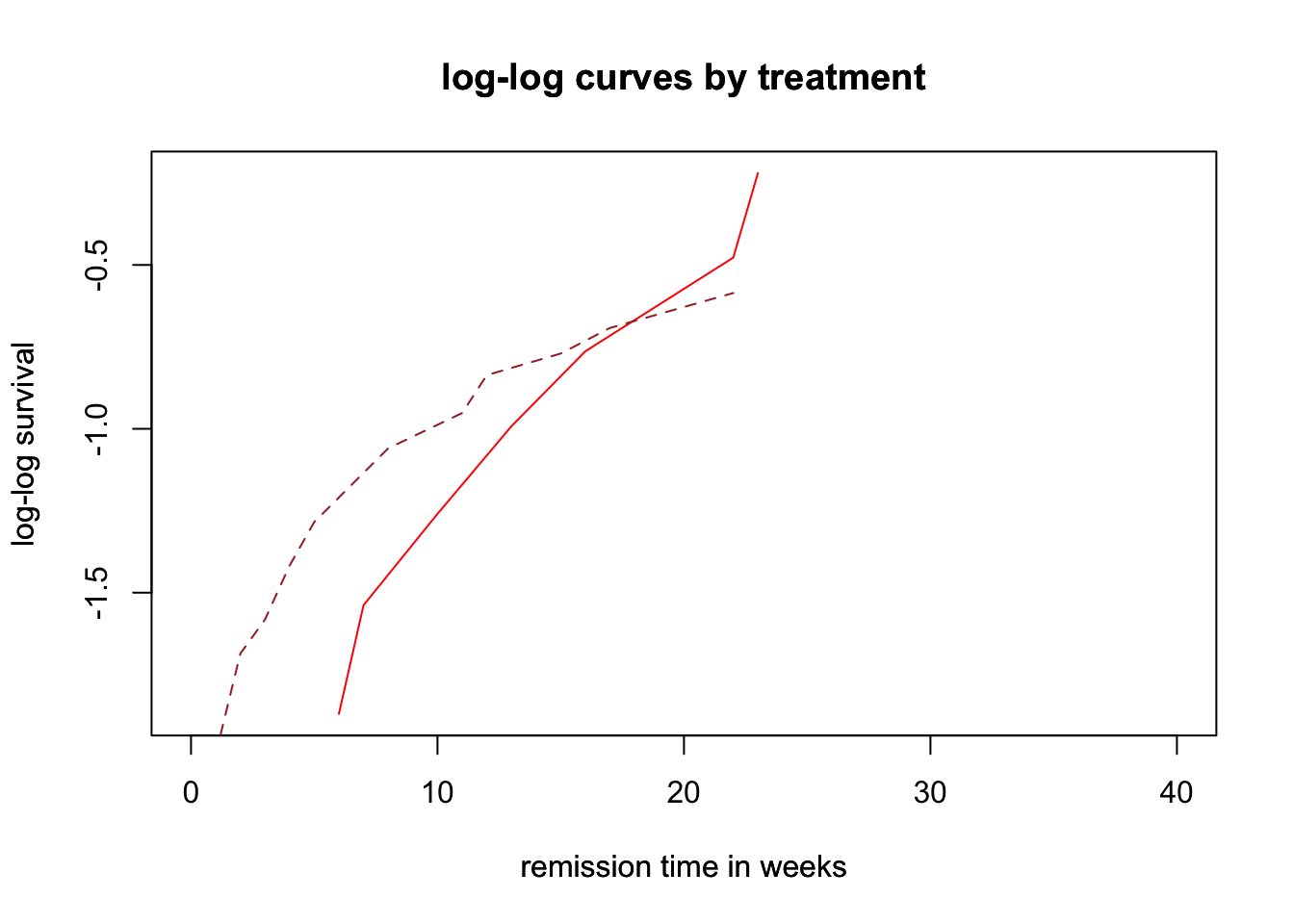From this log(-log(S)) it seems that the proportional hazard model assumption for the treatment covariates seems to be violated, because the parallel assumption is violated. plot observed Kaplan Meier Curve and compare it with survival curve under Cox-PH model The idea here is to compare the Kaplan Meier Curve and the survival curve measured in Cox-PH model. If the Kaplan Meier Curve is different from the survival Cox-PH model, then the PH assumption is violated. plot_1=plot(surv_model_3,lty=c("solid","dashed"),col=c("black","blue"),xlab="remission time in days",ylab="survival_probability") legend("topright",c("6-MP","Placebo"),lty=c("solid","dashed"),col=c("black","blue"))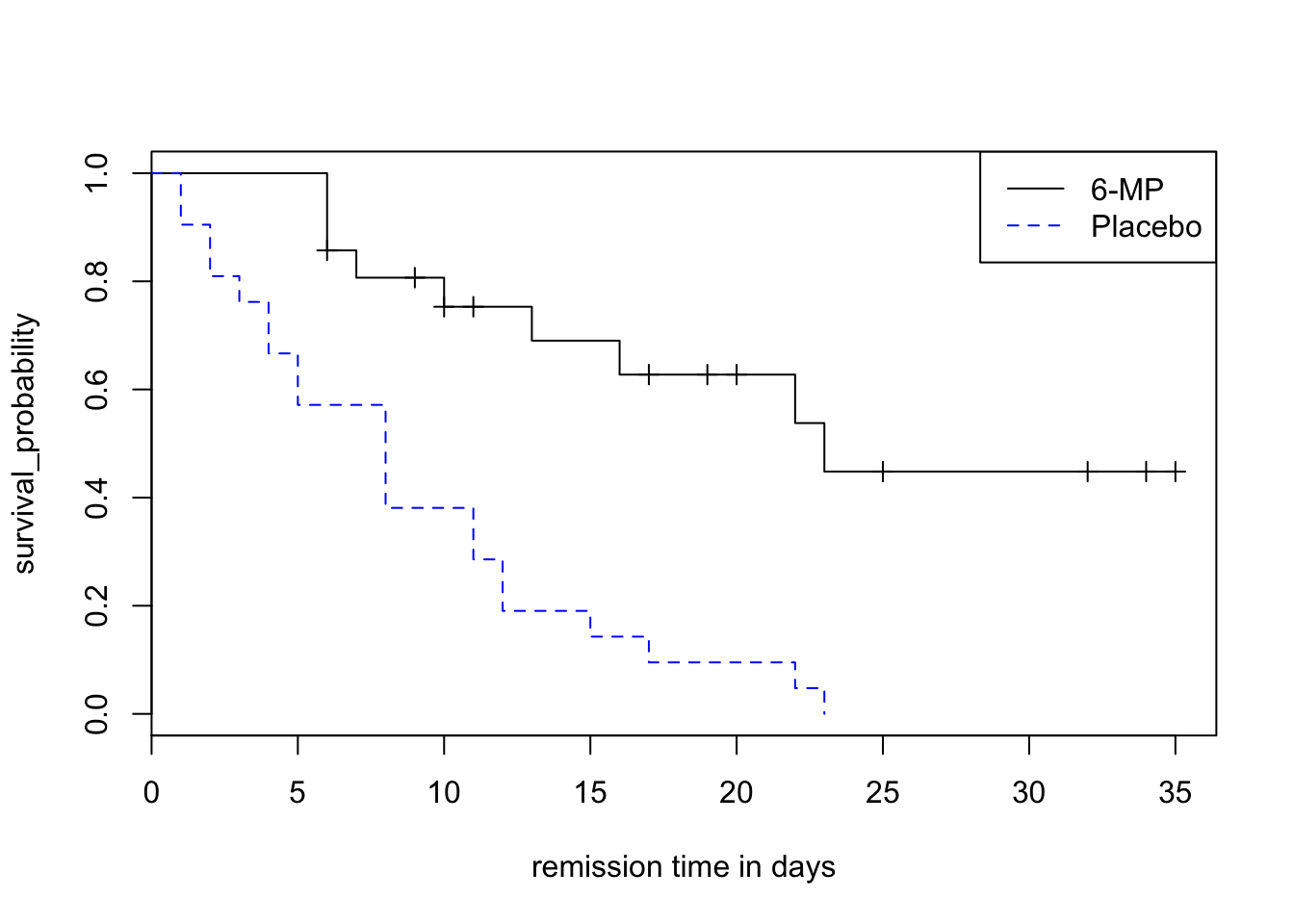plot_2=plot(surv_fit_1,lty=plotstuff$line,col=plotstuff$colour) legend("topright",legend=surv_new$Treatment,title="treatment",lty=plotstuff$line,col=plotstuff$col)
## Warning: Name partially matched in data frame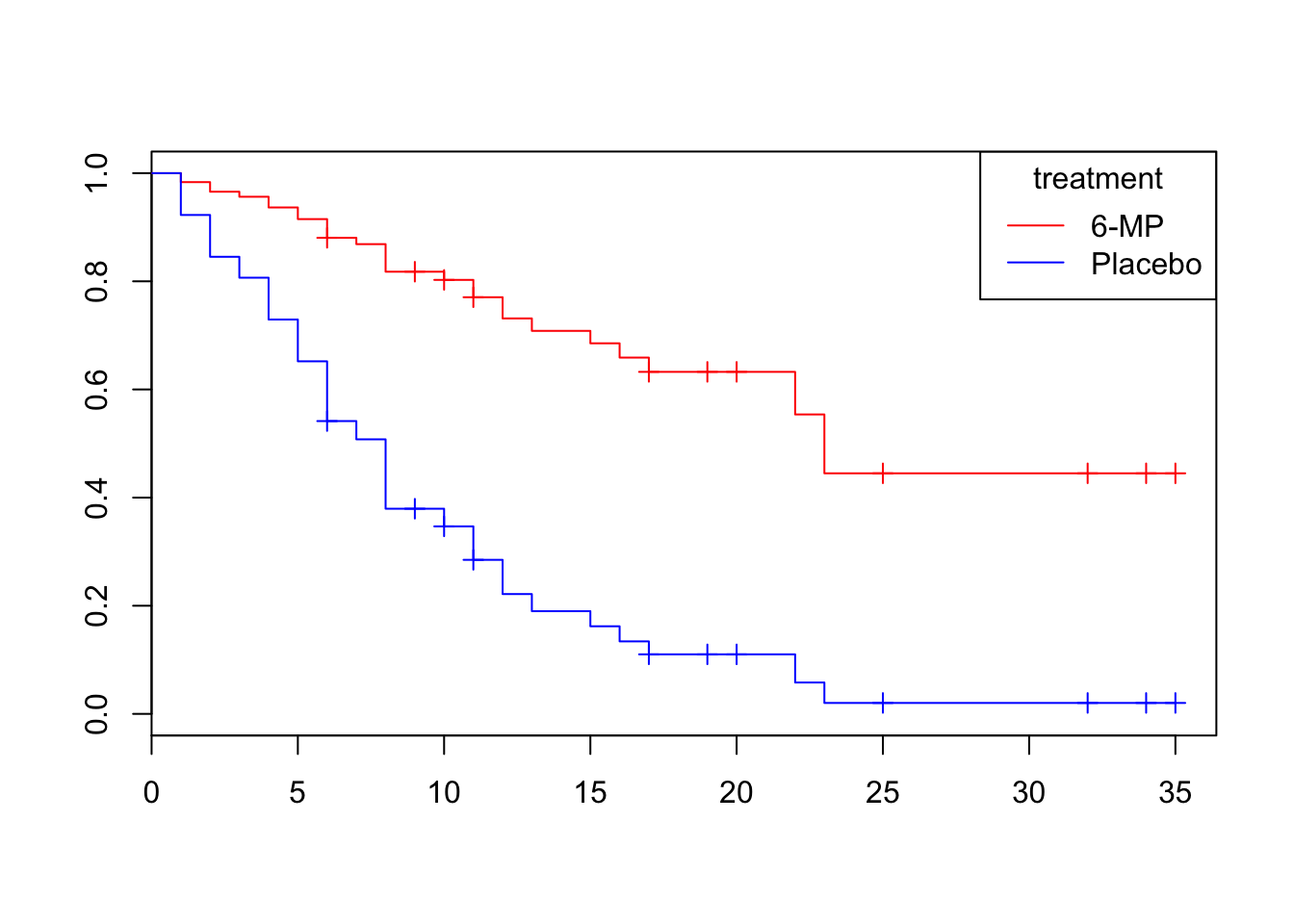In this Case, the survival curve and Kaplan Meier Curve seems to be close, therefore the treatment group complies with PH assumption.

It is Interesting to see that sometimes log-log curves and plot observed vs expected values can give a different result. Since graphical test is very subjective, it is important for the Statistician to develop a formal statistical test.

## Goodness of Fit Test:

The Goodness of Fit Test is an objective testing, because it provides a test statistic and p-value for assessing the PH assumption for a given predictor of interest. A number of different test statistic were proposed by different researcher, but the common method of to do Goodness of Fit Test is to take a look at Schoenfeld Residuals. The idea here is that if the PH assumption holds for a particular covariates, then its schoenfeld residual for that covariate will not be related to survival time.

Therefore, it is a way to check the correlation between schoenfeld residual vs survival time. If the correlation is not equal to 0, therefore the PH assumption is violated.

cox.zph(surv_model,transform=rank)
##                      rho  chisq     p
## TreatmentPlacebo -0.0296 0.0258 0.872

Since the P-value of Schoenfeld residual test is high, therefore the treatment covariate complies with the cox-proportional hazard assumption.

# Stratified Cox Model

Stratifited Cox Model is a modification of CoxPH model that allows for control by stratification of predictor not satisfying PH assumption. The idea is to run Cox Proportional hazard model on the variables that satisfy Cox PH assumption under the strata of the variables not satisfy Cox PH assumption.

General Stratified Cox Model

Suppose that we have k variables that do not satisfy PH assumption denoted by Z1,Z2,…,Zk and p variables satisfy PH assumption X1,X2,X3,…,Xp. To perform the stratified cox procedure, we define a single Z * from the Z used for stratification. Therefore Z has k * categories, where k * is the total # of combinations of strata.

Hazard Function of General Stratified Cox Model: $$\mbox h_g (t,X) = h_{0g} (t) * exp(\beta_1*X1+\beta_2*X2+...+\beta_p*Xp)$$

In this case, the Z* variable that does not follow PH assumption is not included in the model. Unlike before, the baseline hazard function will be different for each of the strata. i.e $$\mbox h_{01} (t) != h_{02} (t) != h_{03} (t) !=.... != h_{0g} (t)$$

but the $$\beta's$$ is the same for every strata. To obtain the estimate of for the $$\beta's$$ we maximized the partial likelihood function that was obtained by multiplying likelihood functions together.

No Interaction Assumption of SC

Stratified Cox Model $$h_g(t,X) = h_{og} (t)*exp(\beta_1*X1+\beta_2*X2+....+\beta_p*Xp)$$

In this case, $$(\beta_1,\beta_2,....,\beta_p)$$ contains the same value for each of the strata.

Interaction model by fitting separate models

$$h_g(t,X)= h_{og} (t)*exp(\beta_{1g}*X1+\beta_{2g}*X2+....+\beta_{ng}*Xn)$$ where g = 1 for one classification g=2 for another classification of strata.

note that $$(\beta_{1g},\beta_{2g},....,\beta_{ng})$$ contains different value for each of the strata variable.

Alternative Interaction Model:

$h_g(t,X)= h_{og} (t)*exp(\beta_{1}*X1+\beta_{2}*X2+....+\beta_{g}*Xg+\beta_{11}*(Z1*X1)+\beta_{12}$

$*(Z2*X1)+...+\beta_{1g}(Zg*X1)+\beta_{21}*(Z1*X2)+\beta_{22}*(Z2*X2)+....+\beta_{2g}$

$*(Zg*X2)+........+\beta{g1}*(Zg*X1)+.....+\beta{gg}*(Zg*Xg)$

This model is extremely complicated. However, there is some sort of manipulation that can be done to equate both Interaction Model.

The Example of Stratified Cox Model from Chapter 5 Test Question:

Suppose that we are trying to analyze time of days spent by heroin addicts from entry to departure from one of the two methadone clinics, the other two covariates were also taken into consideration(prison record and methadone dose)

#An Attempt to do Statistical Analysis for the Test Question from Chapter 5
head(addicts)
##   V1 V2 V3  V4 V5 V6
## 1  1  1  1 428  0 50
## 2  2  1  1 275  1 55
## 3  3  1  1 262  0 55
## 4  4  1  1 183  0 30
## 5  5  1  1 259  1 65
## 6  6  1  1 714  0 55
names(addicts)= c("ID","clinic","surv_status","surv_time","prison","methadone_dose")
library(splines)
library(survival)
response=Surv(addicts$surv_time,addicts$surv_status)
explanatory_var=cbind(addicts$clinic,addicts$prison,addicts$methadone_dose) #explanatory_var #no interaction model addict_cox=coxph(response~addicts$clinic+addicts$prison+addicts$methadone_dose)
#interaction model
addict_cox_2=coxph(response~addicts$clinic+addicts$prison+addicts$methadone_dose+addicts$clinic*addicts$prison+addicts$clinic*addicts$methadone_dose) addict_cox ## Call: ## coxph(formula = response ~ addicts$clinic + addicts$prison + ## addicts$methadone_dose)
##
##
##                           coef exp(coef) se(coef)     z       p
## addicts$clinic -1.0099 0.364 0.21489 -4.70 2.6e-06 ## addicts$prison          0.3266     1.386  0.16722  1.95 5.1e-02
## addicts$methadone_dose -0.0354 0.965 0.00638 -5.54 2.9e-08 ## ## Likelihood ratio test=64.6 on 3 df, p=6.23e-14 n= 238, number of events= 150 addict_cox_2 ## Call: ## coxph(formula = response ~ addicts$clinic + addicts$prison + ## addicts$methadone_dose + addicts$clinic * addicts$prison +
##     addicts$clinic * addicts$methadone_dose)
##
##
##                                          coef exp(coef) se(coef)      z
## addicts$clinic 0.1796 1.197 0.8933 0.201 ## addicts$prison                         1.1924     3.295   0.5414  2.202
## addicts$methadone_dose -0.0192 0.981 0.0194 -0.990 ## addicts$clinic:addicts$prison -0.7383 0.478 0.4315 -1.711 ## addicts$clinic:addicts$methadone_dose -0.0140 0.986 0.0143 -0.974 ## p ## addicts$clinic                        0.840
## addicts$prison 0.028 ## addicts$methadone_dose                0.320
## addicts$clinic:addicts$prison         0.087
## addicts$clinic:addicts$methadone_dose 0.330
##
## Likelihood ratio test=68.2  on 5 df, p=2.45e-13  n= 238, number of events= 150
names(addict_cox_2)
##   "coefficients"      "var"               "loglik"
##   "score"             "iter"              "linear.predictors"
##   "residuals"         "means"             "concordance"
##  "method"            "n"                 "nevent"
##  "terms"             "assign"            "wald.test"
##  "y"                 "formula"           "call"
#check for violation of proportional hazard
res_addict_cox
##                            rho chisq        p
## addicts$clinic -0.2578 11.19 0.000824 ## addicts$prison         -0.0382  0.22 0.639369
## addicts$methadone_dose 0.0724 0.70 0.402749 ## GLOBAL NA 12.62 0.005546 plot(res_addict_cox)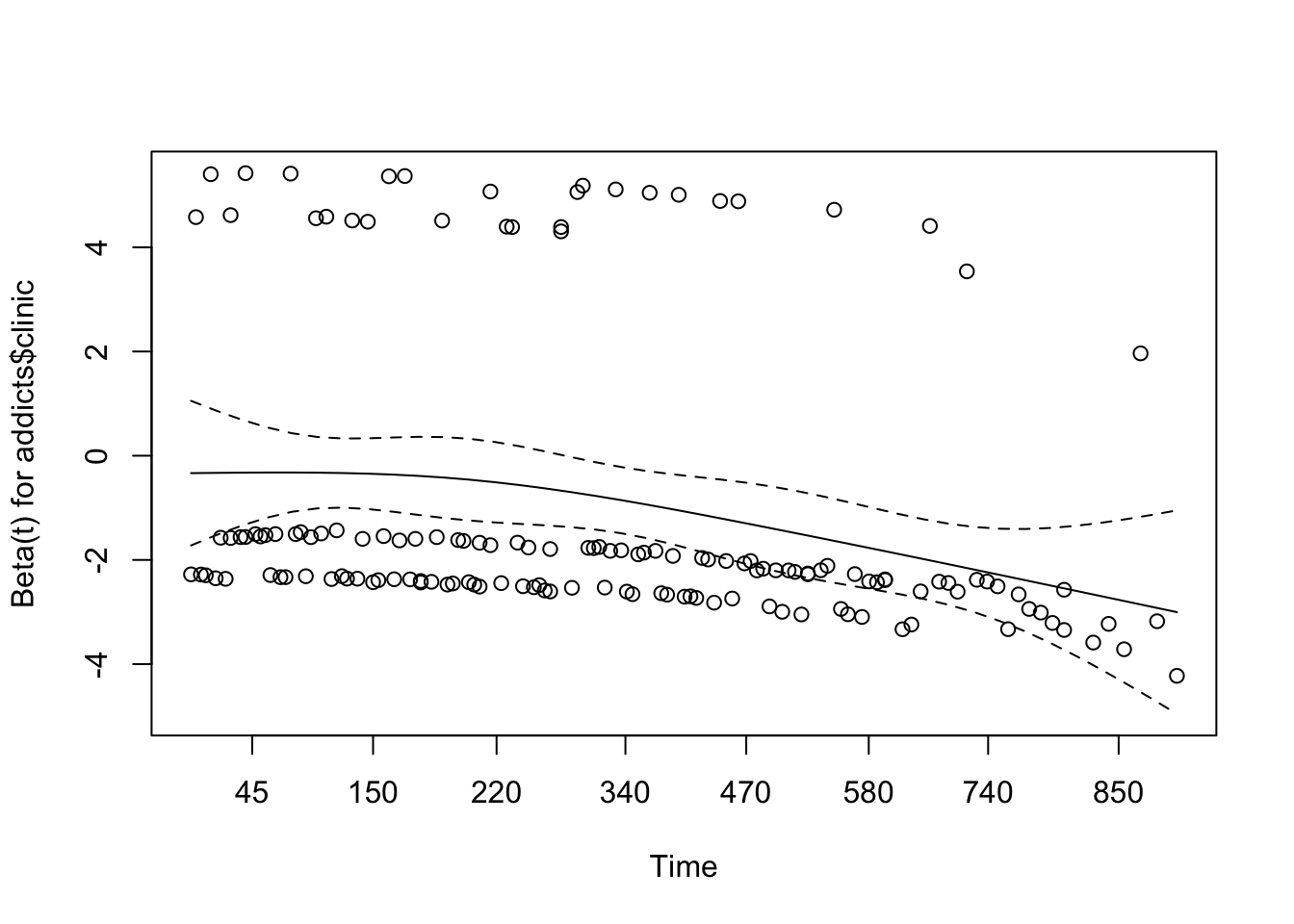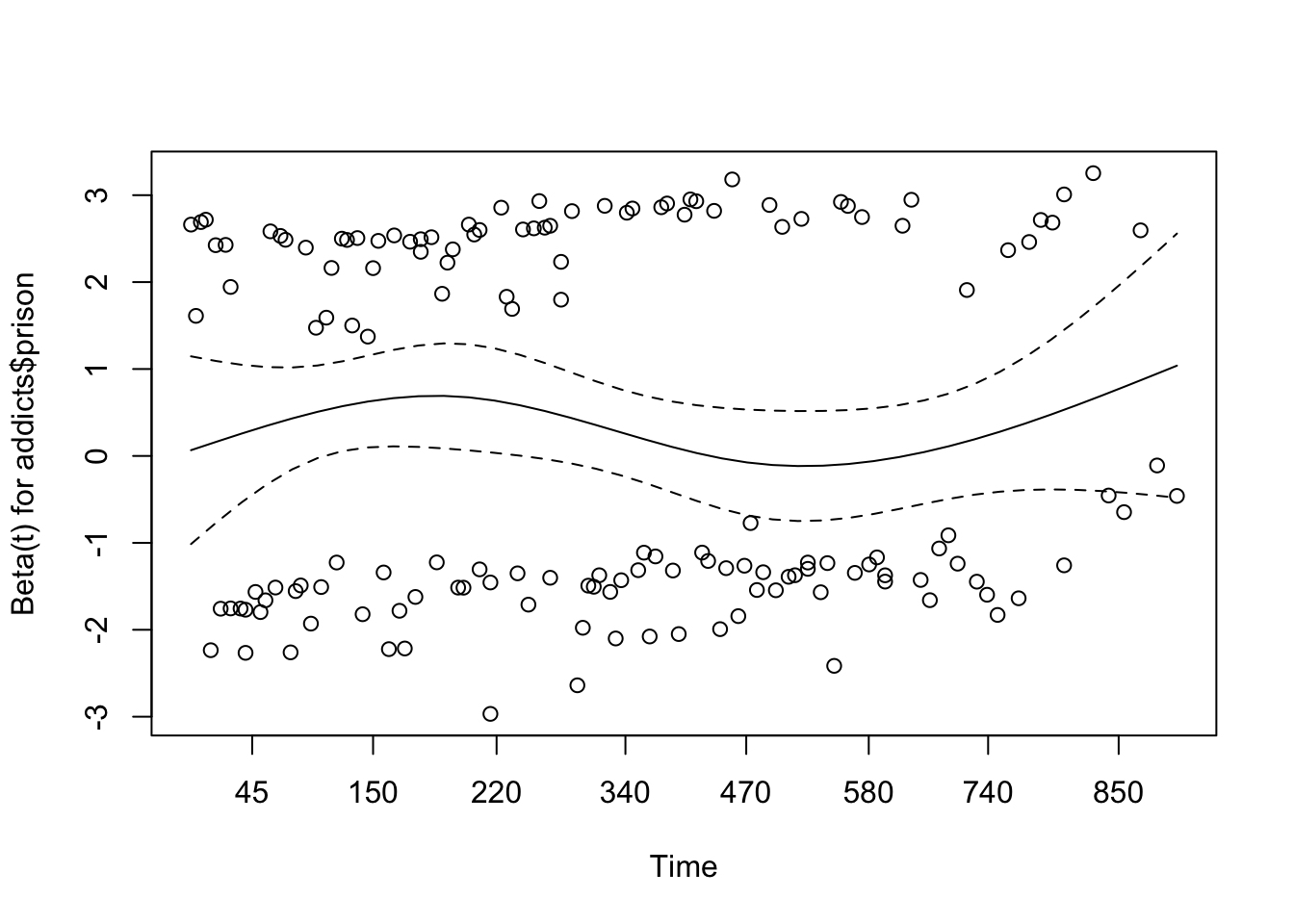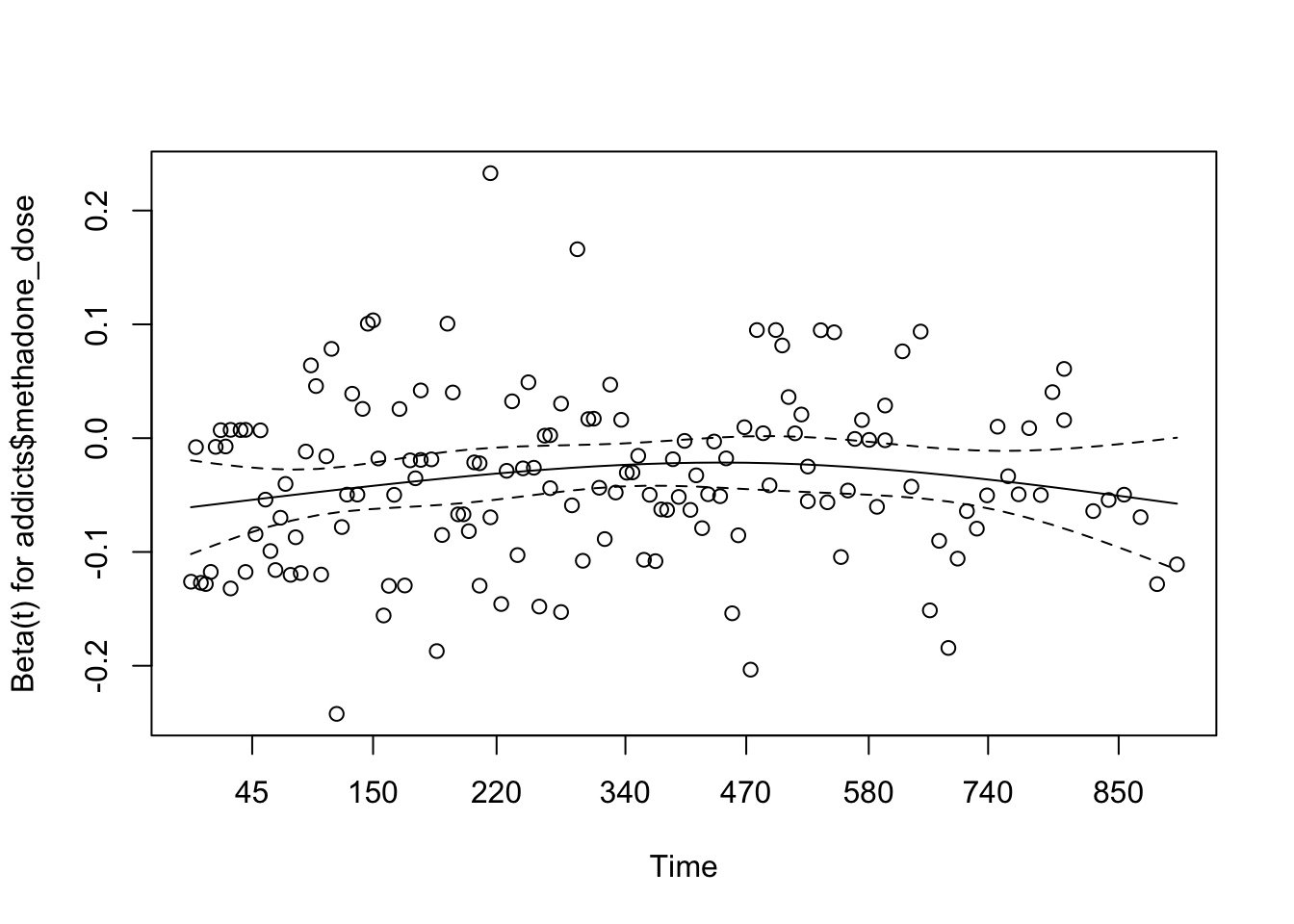residuals(res_addict_cox, type = "scaledsch") ## NULL addict_cox_strata=coxph(response~strata(addicts$clinic)+addicts$prison+addicts$methadone_dose)
summary(addict_cox_strata)
## Call:
## coxph(formula = response ~ strata(addicts$clinic) + addicts$prison +
##     addicts$methadone_dose) ## ## n= 238, number of events= 150 ## ## coef exp(coef) se(coef) z Pr(>|z|) ## addicts$prison          0.38960   1.47640  0.16893  2.31    0.021 *
## addicts$methadone_dose -0.03511 0.96549 0.00646 -5.43 5.6e-08 *** ## --- ## Signif. codes: 0 '***' 0.001 '**' 0.01 '*' 0.05 '.' 0.1 ' ' 1 ## ## exp(coef) exp(-coef) lower .95 upper .95 ## addicts$prison             1.476      0.677     1.060     2.056
## Score (logrank) test = 33.3  on 2 df,   p=5.77e-08
res_addict_cox_2=cox.zph(addict_cox_strata)
plot(res_addict_cox_2)